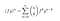# A brief introduction to Taylor series

Today marks the day where I finally do it. The day that I explain the most powerful and wonderful mathematical formula birthed from calculus. What I feel is personally one of the most beautiful ideas I think the human mind has ever conceived. This beautiful Idea is known as the Taylor series. The Taylor series is a method of turning non polynomial functions into polynomial functions. When I say functions, I mean all of the nice kinds;-trigonometric, exponential, logarithmic. Now, this all probably sounds too good to be true and you may scratch your head in disbelief and ask yourself…

# Abstract

Following from the previous article I had written on the Taylor series here, in this article, I present a method for deriving the Leibniz product rule from Taylor’s theorem and Cauchy product rule.

# Introduction

The Leibniz rule gives us a nice closed form for the nth derivative of the product of two functions as a summation of binomial coefficients and derivatives of the individual functions:The exponent denotes derivatives, so the ’n’ on the left side means the nth derivative of the product

Almost looks like a binomial theorem for expanding derivatives, doesn’t it? I wonder if there is a math field that studies sp00ky relationships such as these.

# In problem-solving,

There is a preconceived notion that mathematics is a subject for the gifted or very intelligent, that the whole idea is having hundreds of equations on a blackboard with symbols flying here and there. I’ll tell you here, that’s it not. When I think of mathematics, there are two parts for me: the idea and the execution.

The idea is the principle behind what I’m trying to accomplish and the execution is bashing the problem with compositions of known methods and tools.

When my study of mathematics began, I used to make a lot of mistakes in my calculations (…

# Summing the discrete using Calculus[Beauty of Geometric series part-2]

Last time we calculated sums finite and infinite, applied calculus and counted using the geometric series. This time we will learn how we can apply derivatives onto the finite geometric series for evaluating other discrete sums. The main prerequisites of this article would be knowledge of the binomial theorem and basic differential calculus.

We start by considering a finite geometric progression of the form,